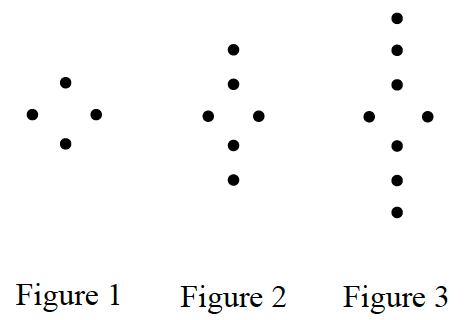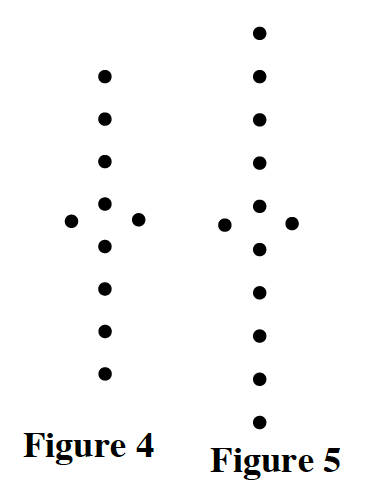### Home > CC1 > Chapter 1 > Lesson 1.2.4 > Problem1-91

1-91.

Study the dot pattern below. 1-91 HW eTool (CPM). Homework Help ✎1. Sketch the $4^{\text{th}}$ and $5^{\text{th}}$ figures.

What is the pattern of the first $3$ figures? Follow this pattern in sketching Figures $4$ and $5$

See Figures 4 and 5 below.2. How many dots will the $50^{\text{th}}$ figure have?

Try finding a way to describe how you add dots to the figures.